## How to Calculate and Solve for Electron Kinetic Energy | X-Ray Crystallography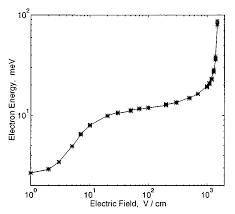The image above represents electron kinetic energy.

To compute for electron kinetic energy, two essential parameters are needed and these parameters are Mass (m) and Velocity (v).

The formula for calculating electron kinetic energy:

eV = mv²/2

Where:

eV = Electron Kinetic Energy
m = Mass
v = Velocity

Let’s solve an example;
Find the electron kinetic energy when the mass is 12 and velocity is 24.

This implies that;

m = Mass = 12
v = Velocity = 24

eV = mv²/2
eV = (12)(24)²/2
eV = (12)(576)/2
eV = (6912)/2
eV = 3456

Therefore, the electron kinetic energy is 3456 J.

Calculating the Mass when the Electron Kinetic Energy and the Velocity is Given.

m = eV x 2 / v2

Where;

m = Mass
eV = Electron Kinetic Energy
v = Velocity

Let’s solve an example;
Find the mass when the electron kinetic energy is 12 and the velocity is 4.

This implies that;

eV = Electron Kinetic Energy = 12
v = Velocity = 4

m = eV x 2 / v2
m = 12 x 2 / 42
m = 24 / 16
m = 1.5

Therefore, the mass is 1.5.

## How to Calculate and Solve for Velocity | De Broglie’s Law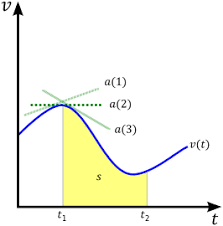The image above represents velocity.

To compute for velocity, three essential parameters are needed and these parameters are Planck’s Constant (h), Wavelength (λ) and Mass (m).

The formula for calculating velocity:

v = h / λm

Where;

v = velocity
λ = wavelength
h = Planck’s constant
m = mass

Let’s solve an example;
Find the velocity when the wavelength is 10, the planck’s constant is 6.626e-34 and the mass is 5.

This implies that;

λ = wavelength = 10
h = Planck’s constant = 6.626e-34
m = mass = 5

v = h / λm
v = 6.62607004e-34 (10)(5)
v = 6.62607004e-34 (50)
v = 1.33e-35

Therefore, the velocity is 1.33e-35 m/s.

## How to Calculate and Solve for Mass | De Broglie’s Law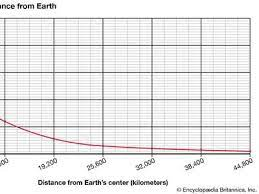The image above represents mass.

To compute for mass, three essential parameters are needed and these parameters are Planck’s Constant (h), Wavelength (λ) and Velocity (v).

The formula for calculating the mass:

m = h / λv

Where;

m = mass
λ = wavelength
h = Planck’s constant
v = velocity

Let’s solve an example;
Find the mass when the wavelength is 4, the planck’s constant is 6.626e-34 and the velocity is 2.

This implies that;

λ = wavelength = 4
h = Planck’s constant = 6.626e-34
v = velocity = 2

m = hλv
m = 6.62607004e-34(4)(2)
m = 6.62607004e-34(8)
m = 8.28e-35

Therefore, the mass is 8.28e-35 kg.

## How to Calculate and Solve for Wavelength | De Broglie’s Law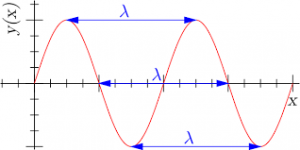The image above represents wavelength.

To compute for wavelength, three essential parameters are needed and these parameters are Planck’s Constant (h), Mass (m) and Velocity (v).

The formula for calculating wavelength:

λ = hmv

Where

λ = wavelength
h = Planck’s constant
m = mass
v = velocity

Let’s solve an example;
Find the wavelength when the planck’s constant is 6.62e-34, the mass is 21 and the velocity is 11.

This implies that;

h = Planck’s constant = 6.62e-34
m = mass = 21
v = velocity = 11

λ = hmv
λ = 6.62607004e-34(21)(11)
λ = 6.62607004e-34(231)
λ = 2.86e-36

Therefore, the wavelength is 2.86e-36 m.

Calculating the Mass when the Wavelength, the Planck’s Constant and the Velocity is Given.

m = h / λv

Where;

m = mass
λ = wavelength
h = Planck’s constant
v = velocity

Let’s solve an example;
Find the mass when the wavelength is 18, the planck’s constant is 6.626e-34 and the velocity is 6.

This implies that;

λ = wavelength = 18
h = Planck’s constant = 6.626e-34
v = velocity = 6

m = h / λv
m = 18 / 6.626e-34 x 6
m = 18 / 3.976e-33
m = 4.53

Therefore, the mass is 4.53 m.

## How to Calculate and Solve for Linear Momentum | Motion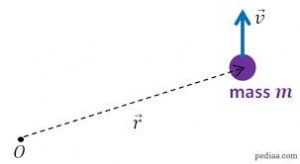The image above represents linear momentum.

To compute for linear momentum, two essential parameters are needed and these parameters are mass (m) and velocity (v).

The formula for calculating linear momentum:

p = mv

Where;

p = Momentum
m = Mass
v = Velocity

Let’s solve an example;
Find the momentum when the mass is 8 and the velocity is 12.

This implies that;

m = Mass = 8
v = Velocity = 12

p = mv
p = 8 x 12
p = 96

Therefore, the momentum is 96 Kgm/s.

Calculating the Mass when the Momentum and the Velocity is Given.

m = p / v

Where;

m = Mass
p = Momentum
v = Velocity

Let’s solve an example;
Find the mass when the momentum is 40 and the velocity is 18.

This implies that;

p = Momentum = 40
v = Velocity = 18

m = p / v
m = 40 / 18
m = 2.22

Therefore, the mass is 2.22 kg.

## How to Calculate and Solve for Velocity | MotionThe image above represents velocity.

To compute for velocity, three essential parameters are needed and these parameters are angular velocity (ω), amplitude (A) and displacement (y).

The formula for calculating velocity:

v = ω√(A² – y²)

Where;

v = Velocity
ω = Angular Velocity
A = Amplitude
y = Displacement

Let’s solve an example;
Find the velocity when the angular velocity is 7, amplitude is 10 and displacement is 13.

This implies that;

ω = Angular Velocity = 7
A = Amplitude = 10
y = Displacement = 13

v = ω√(A² – y²)
v = 7 x √(10² – 13²)
v = 7 x √(100 – 169)
v = 7 x √(-69)

i signifies a complex number = √(-1).

v = 7 x √(69) x √(-1)
v = 7 x √(69)i
v = 7 x 8.30i
v = 7(8.30i)

Therefore, the velocity is 7(8.30i) m/s.

Calculating the Angular Velocity when the Velocity, the Amplitude and the Displacement is Given.

ω = v / √(A2 – y2)

Where;

ω = Angular Velocity
v = Velocity
A = Amplitude
y = Displacement

Let’s solve an example;
Find the angular velocity when the velocity is 28, the amplitude is 14 and the displacement is 10.

This implies that;

v = Velocity = 28
A = Amplitude = 14
y = Displacement = 10

ω = v / √(A2 – y2)
ω = 28 / √(142 – 102)
ω = 28 / √(196 – 100)
ω = 28 / √(96)
ω = 28 / 9.79
ω = 2.86

Therefore, the angular velocity is 2.86.

## How to Calculate and Solve for Velocity | Motion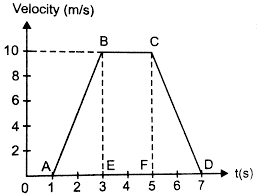The image above represents velocity.

To compute for velocity, two essential parameters are needed and these parameters are angular velocity (ω) and radius (r).

The formula for calculating velocity:

v = ωr

Where;

v = Velocity
ω = Angular Velocity

Let’s solve an example;
Find the velocity when the angular velocity is 17 with a radius of 6.

This implies that;

ω = Angular Velocity = 17

v = ωr
v = 17 x 6
v = 102

Therefore, the velocity is 102 m/s.

Calculating the Angular Velocity when the Velocity and the Radius is Given.

ω =v / r

Where;

ω = Angular Velocity
v = Velocity

Let’s solve an example;
Given that the velocity is 30 with a radius of 3. Find the angular velocity?

This implies that;

v = Velocity = 30

ω = v / r
ω = 30 / 3
ω = 10

Therefore, the angular velocity is 10.

## How to Calculate and Solve for Maximum Velocity to avoid Overturning of a Vehicle moving along a Level Circular Path | The Calculator Encyclopedia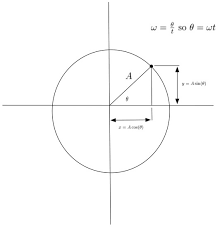The image above represents the maximum velocity to avoid overturning of a vehicle moving along a level circular path.

To compute for the maximum velocity, four essential parameters are needed and these parameters are Acceleration due to Gravity (g), Height of Centre of Gravity of the Vehicle from Ground Level (h), Radius of Circular Path (r) and Half of the Distance between the Centre Lines of the Wheel (a).

The formula for calculating the maximum velocity:

vmax = √(gra / h)

Where:
vmax = Maximum Velocity to avoid Overturning of a Vehicle moving along a Level Circular Path
g = Acceleration due to Gravity
h = Height of Centre of Gravity of the Vehicle from Ground Level
r = Radius of Circular Path
a = Half of the Distance between the Centre Lines of the Wheel

Let’s solve an example;
Find the maximum velocity when the Acceleration due to Gravity (g) is 10.2, Height of Centre of Gravity of the Vehicle from Ground Level (h) is 14, Radius of Circular Path (r) is 22 and Half of the Distance between the Centre Lines of the Wheel (a) is 32.

This implies that;
g = Acceleration due to Gravity = 10.2
h = Height of Centre of Gravity of the Vehicle from Ground Level = 14
r = Radius of Circular Path = 22
a = Half of the Distance between the Centre Lines of the Wheel = 32

vmax = √(gra / h)
vmax = √((10.2)(22)(32)/14)
vmax = √((7180.79)/14)
vmax = √(512.91)
vmax = 22.647

Therefore, the maximum velocity to avoid Overturning of a Vehicle moving along a Level Circular Path is 22.647 m/s.

## How to Calculate and Solve for the Reaction at the Inner Wheel of a Vehicle moving along a Level Circular Path | The Calculator Encyclopedia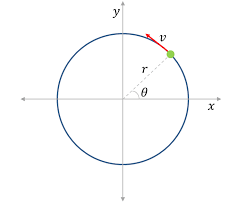The image represents reaction at the inner wheel of a vehicle moving along a level circular path.

To compute for the reaction, six essential parameters are needed and these parameters are Mass of the Vechicle (m), Acceleration due to Gravity (g), Velocity of the Vehicle (v), Height of Centre of Gravity of the Vehicle from Ground Level (h), Radius of Circular Path (r) and Half of the Distance between the Centre Lines of the Wheel (a).

The formula for calculating the reaction at the inner wheel of a vehicle moving along a level circular path:

RA = mg / 2[1 – v²h / gra]

Where:
RA = Reaction at the Inner Wheel of a Vehicle moving along a Level Circular Path
m = Mass of the Vechicle
g = Acceleration due to Gravity
v = Velocity of the Vehicle
h = Height of Centre of Gravity of the Vehicle from Ground Level
r = Radius of Circular Path
a = Half of the Distance between the Centre Lines of the Wheel

Let’s solve an example;
Find the reaction when Mass of the Vechicle (m) is 13, Acceleration due to Gravity (g) is 9.8, Velocity of the Vehicle (v) is 11, Height of Centre of Gravity of the Vehicle from Ground Level (h) is 5, Radius of Circular Path (r) is 7 and Half of the Distance between the Centre Lines of the Wheel (a) is 3.

This implies that;
m = Mass of the Vechicle = 13
g = Acceleration due to Gravity = 9.8
v = Velocity of the Vehicle = 11
h = Height of Centre of Gravity of the Vehicle from Ground Level = 5
r = Radius of Circular Path = 7
a = Half of the Distance between the Centre Lines of the Wheel = 3

RA = mg / 2[1 – v²h / gra]
RA = 13(9.8) / 2[1 – (11)²(5) / (9.8)(7)(3)]
RA = 127.4 / 2[1 – (121)(5) / 205.8]
RA = 63.7[1 – 605 / 205.8]
RA = 63.7[1 – 2.939]
RA = 63.7[-1.939]
RA = -123.56

Therefore, the reaction at the inner wheel of a vehicle moving along a level of circular path is -123.56 N.

## How to Calculate and Solve for Road Bank Angle, Velocity and Radius of a Body in Motion of Circular Path | The Calculator Encyclopedia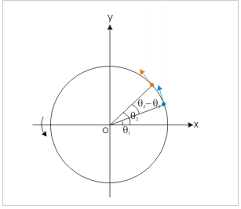The image represents road bank angle in circular motion.

To compute for the road bank angle, three essential parameters are needed and these parameters are velocity (v), acceleration due to gravity (g) and radius (r).

The formula for calculating the road bank angle;

θ = tan-1( / gr)

Where;
v = Velocity
g = Acceleration due to Gravity

Let’s solve an example;
Find the road bank angle where the acceleration due to gravity is 9.8, velocity is 35 and radius is 18.

This implies that;
v = Velocity = 35
g = Acceleration due to Gravity = 9.8

θ = tan-1( / gr)
θ = tan-1(35² / (9.8)(18))
θ = tan-1(1225 / 176.4)
θ = tan-1(6.94)
θ = 81.81°

Therefore, the road bank angle is 81.81°.

Calculating the Velocity when Road Bank Angle, Acceleration due to Gravity and Radius is Given.

v = √gr.tan θ

Where;
v = Velocity
g = Acceleration due to Gravity

Let’s solve an example;
Given that the road bank angle is 50, radius is 15 and acceleration due to gravity is 9.8. Find the velocity?

This implies that;
θ = Road Bank Angle = 50
g = Acceleration due to Gravity = 9.8